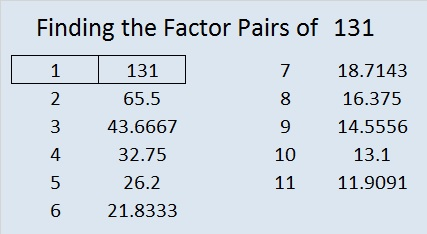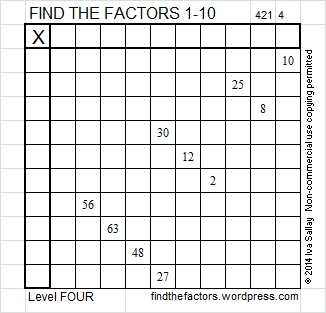# 131 and Level 4

• 131 is a prime number.
• Prime factorization: 131 is prime.
• The exponent of prime number 131 is 1. Adding 1 to that exponent we get (1 + 1) = 2. Therefore 131 has exactly 2 factors.
• Factors of 131: 1, 131
• Factor pairs: 131 = 1 x 131
• 131 has no square factors that allow its square root to be simplified. √131 ≈ 11.4455How do we know that 131 is a prime number? If 131 were not a prime number, then it would be divisible by at least one prime number less than or equal to √131 ≈ 11.4. Since 131 cannot be divided evenly by 2, 3, 5, 7, or 11, we know that 131 is a prime number.

131 is never a clue in the FIND THE FACTORS puzzles.Excel file of puzzles and previous week’s solutions: 10 Factors 2014-05-26## 2 thoughts on “131 and Level 4”

1.Joseph Nebus

Well, that one was harder, but once I got the 6’s squared away things fell into place.

2.ivasallay

There certainly were several places 6’s could have gone. Thanks for solving the puzzles week in and week out!

This site uses Akismet to reduce spam. Learn how your comment data is processed.